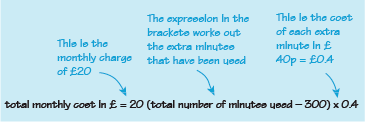Succeed with maths: part 2

Start this free course now. Just create an account and sign in. Enrol and complete the course for a free statement of participation or digital badge if available.

One of the advantages of identifying the general features of a calculation and then describing it mathematically is that the formula can then be used in a computer to work out different calculations quickly and efficiently. Many utility suppliers (gas, water, electricity, telephone) have rates based on a fixed daily charge and a further charge based on how much you have used during the billing period.

For example, a mobile phone network charged £20 per month for 300 minutes (or less) of phone calls. Extra calls above the 300 minutes were charged at 40p per minute.

Assuming that more than 300 minutes were used per month, the formula for the total monthly cost can be expressed by the following word formula:

For simplicity data usage or texting are not included.

In this next activity you’ll unpick how this formula relates to the information given about the way in which bill is worked out. This insight will help when moving onto building your own formulas.

## Activity _unit4.3.3 Activity 8 Understanding the formula

Timing: Allow approximately 10 minutes

Suppose that in one month, 375 minutes of phone calls were made. Explain how you would calculate the cost for the extra minutes (above the 300 minute allowance) and then how to calculate the total cost for that month. Can you then explain how the formula has been put together?

Because 300 minutes are included in the £20 charge, the number of minutes that are charged separately is:

Each extra minute costs 40p. This is the same as saying £0.40 per minute, as . So 75 extra minutes will cost

So the total charge for that month is

The formula says that the fixed charge is £20, and then each minute in excess of the 300 minutes allowed costs 40p. How this information relates to our formula can be shown by including some extra notes with it, as shown in Figure 8:Figure _unit4.3.1 Figure 8 Annotated word formula

Now you know how the formula works, and has been put together (or derived), try applying it in this next activity.

## Activity _unit4.3.4 Activity 9 Mobile Phone bills

Timing: Allow approximately 5 minutes

Use the formula to work out the total monthly cost for the following total number of minutes used:

• a.348 minutes

• a.Substituting 348 for the total number of minutes gives the total cost in pounds as:

So, the bill for the month is £39.20.

Note how this calculation is set out with all the working and a concluding sentence that answers the question precisely. This follows the ideas for correct mathematical communication that were covered at the end of Week 8 in Succeed with Maths Part 1.

• b.250 minutes

• b.The formula only applies if more than 300 minutes of calls are made, so it cannot be used in this case. This is another important step in using formulas – check that they apply in your situation before using them. Here, the charge is £20 for up to 300 minutes of calls, so the charge for this month is £20.

If you have a mobile phone with a similar deal, you could work out a formula that fits yours and use it to check your bills – and get some more practice at the same time. It might also help you to decide if a different deal may suit you better.

Moving on now from everyday formula to those used in academic study, the next section looks at how one set of formulas are used in archaeology to work out the approximate height of some of our ancestors.